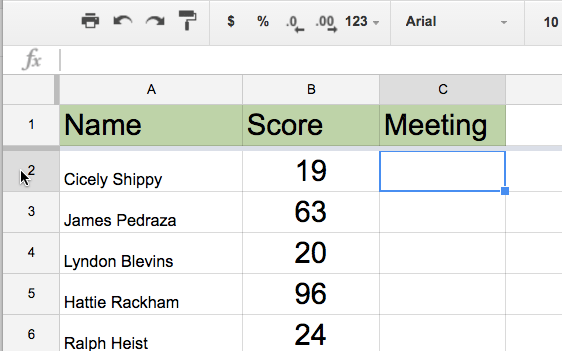# IF Function in Google Sheets

One of my favorite formulas for a spreadsheet is an IF function. If the value of a cell meets certain criteria, do this. Otherwise, do something else.

## If

=IF(criteria, true, false)

## Examples

### Random Sort

I like to use the formula =rand() to assign students a random number and then sort by the random number to choose a student randomly. Doing this allows for the same student to be chosen repeatedly since they are put back in the pool. If you want to draw without replacement, use an IF function.

Create a column to mark off students who have been chosen.In the random column,  you want to assign a random number IF there is no x in the called on column. In my example spreadsheet, I have “Name” in column A, “Called on” in column B, and “Random” in column C. In column C I put

=if(B2=””,rand(),””).

Which says if what is in cell B2 is blank (need double quotations for that), put the formula rand() in cell C2. Otherwise, have C2 be blank (need double quotations for that).### Scores

What if a student has a low score and you want to be alerted to that. If a score is below a certain number you may be concerned about that student. Otherwise, no action.In column C I put

=IF(B2<65,”Concerned”,””)

Which says, if the value in cell B2 is less than 65, put the word concerned in column C. Note that all text strings must be in quotations, so the word Concerned needs to be expressed as “Concerned.” Once again, leaving the cell blank would be double quotations.### Check for Duplicates

I will frequently sort a list of data to see if there are duplicate entries. Manually checking for duplicates is flawed, so I use a formula. First sort the column of data that potentially has repeated information, this puts the repeats next to each other. In another column write an IF statement.

This checks to see if the value in the row above is the same. If it is the same, put the word “ALERT” in column B. Otherwise, do nothing. Click back on the cell with the formula and use the fill down feature to copy the formula to the entire list.## Other Ideas

I get pretty fancy with my IF statements sometimes. For another blog post, I do nested IF statements such as

=IF(A2<60,”F”,IF(A2<70,”D”,IF(A2<80,”C”,IF(A2<90,”B”,”A”))))

How do you use IF statements? Leave your suggestions in the comments below.